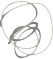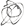Noppanit

07 Jul 2015

# The most basic example of Linear Regression

Just for fun, I wanted to learn how to do linear regression and here’s the example I come up with.

Let’s say you have a historical data of 1000 people who dined in your restaurant and left a tip. This is going to be perfect data because I generated. In the real world you will not find something like this.

If you don’t understand Linear Regression like me before I wrote this post, I recommend you to read this basic linear regression..

The idea is that you have two variables. In this case, it’s tips and total amount of bill. You should explore the data by plotting the graph of these two variables. From my generated data you will get something like this.You can clearly see that there’s a strong correlation between the amount of tip and meal.

Now if you can find the slope of the graph and intercept you should be able to use the formula.

Y = MX + C

M = slope of the graph
C = Intercept


If you’re lazy to look at my notebook.

Then you can run this code.

import pandas as pd
import numpy as np
from scipy import stats

total_bills = np.random.randint(100, size=1000)
tips = total_bills * 0.10

x = pd.Series(tips, name='tips')
y = pd.Series(total_bills, name='total_bills')
df = pd.concat([x, y], axis=1)

slope, intercept, r_value, p_value, std_err = stats.linregress(x=total_bills, y=tips)
predicted_tips = (slope * 70) + intercept


The result is \$7 which corresponds to the 10% tip.

Til next time,
noppanit at 00:00